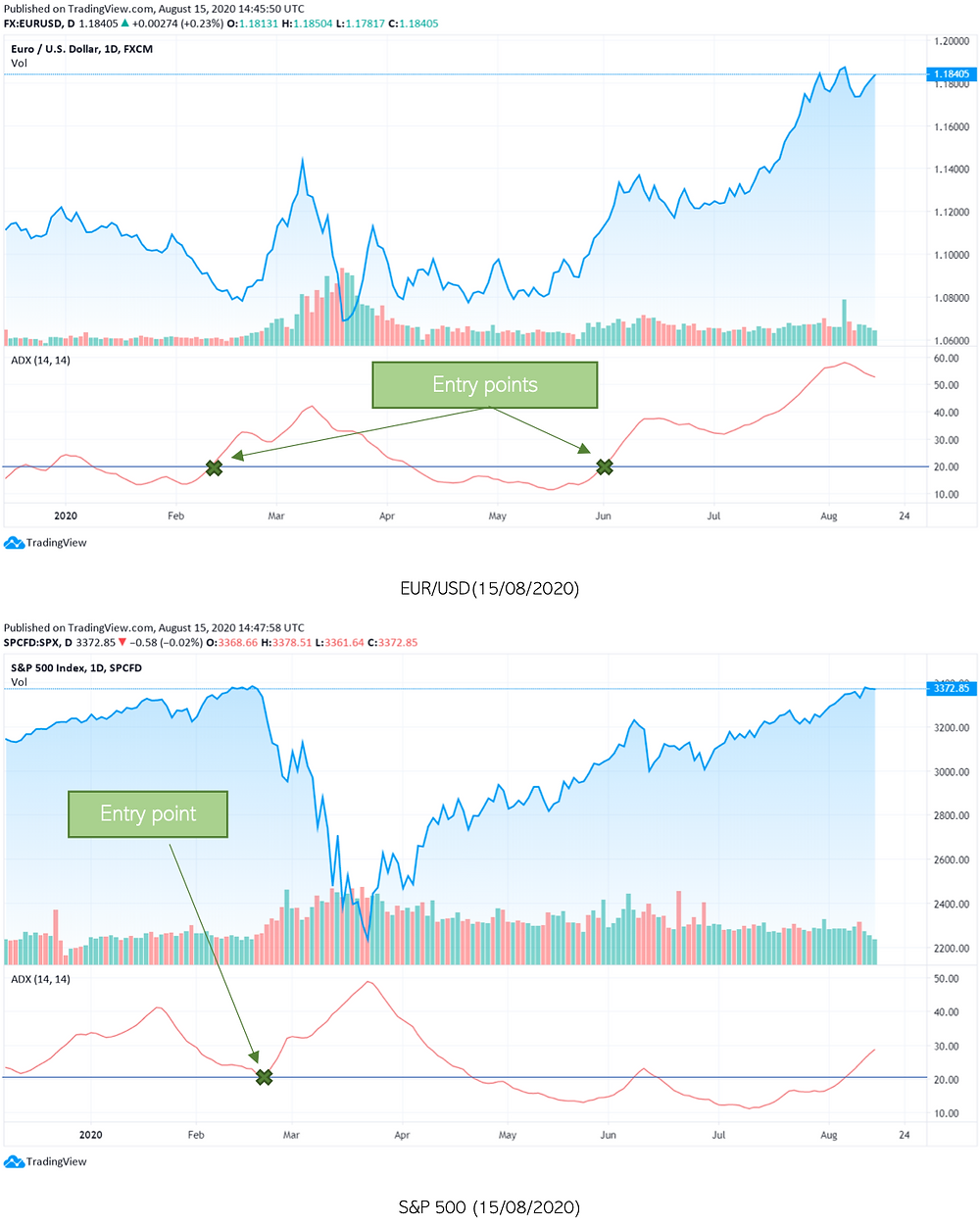Search
• Bocconi Students Options Club

# Average Directional Index

The Average Directional Index (ADX), also called Average Directional Movement Index, is a technical indicator used to determine the strength of a trend. It is made of some minor sub-indicators that, combined, allow the calculation and determination of the strength of a bull or bear market.

### Who invented it?

The ADX was invented and developed in 1978 by J. Welles Wilder. Born in the 1930s Mr. Wilder was a mechanical engineer who became a real estate developer and later on a technical analyst. He designed some of the most common technical indicators used today such as the Average True Range, Relative Strength Index (RSI), Parabolic SAR, the Average Directional index and many more.

### How does it Work?

The Average Directional Index makes use of two sub-indicators: +DI (or positive directional indicator) and -DI (or negative directional indicator). These are used to calculate the DX (or directional index) line, which gives (combined with the DX of the previous periods) the ADX. This indicator is shown on a graph that ranges from 0 to 100 and its level determines the strength of a trend. Therefore, it is important to note that the ADX does not show the trend itself (whether it’s positive or negative).

### The Mathematics Behind

As previously mentioned, to calculate the ADX- its components need to be calculated first. The formulae are:The 2 lines -DI and +DI are momentum indicators, which represent in the graph the trend direction. The ADX is used to understand when to possibly enter or exit a trade and it produces two types of signals: one originated by the level of the ADX (from 0 to 100) and the other by +DI/-DI crossovers.

Firstly, we can identify some reference levels on the charts. For example, 20 is a widely used level. If the ADX is below this horizontal line (25 or 20 level), market directional strength is low and the asset's price is trendless. Per contra, if we find ourselves above this threshold the direction is likely to continue as the current trend is gaining power. When the ADX crosses the 20-25 level it generates a signal, that could suggest entering long or short positions.

The second type of signals produced are the -DI and +DI crossovers. In this case, for example, if the -DI crosses above the +DI it might create an opportunity to enter a potential short trade and vice versa. Most importantly, these types of signals need to take into account the current ADX level, as crossovers at <20 ADX level are false signals (as the asset remains trendless).

Let’s now have a look at how an ADX graph looks like and how it applies:### ExamplesFrom the examples above, we can clearly see three points in which the strength of the trend was growing. In the case of the EUR/USD exchange this happened with a positive price tendency just as for the S&P-500 before the Covid-19 dip of March.

This indicator is, thus, very useful as it allows the user to understand more about the current trend and its strength. It can also be used to know how long a position should be kept open, as a strength decrease could suggest closing it. Therefore, combining this oscillator with others can help developing a complete toolkit for efficient technical analysis.

See All